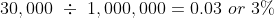# Rental Property Calculator

Purchase
attach_money
attach_money
No
%
%
Years
Income
attach_money
%
Operating Expenses
attach_money
attach_money
attach_money
attach_money

### Rental Income

Gross Rental Income is the total amount of money you will get from renting out your property without accounting for costs or expenses. It is calculated by multiplying the monthly rent by 12 (i.e. 1 year) and then factoring in the vacancy rate. The vacancy rate(%) is the amount of time your property is empty and not making money. Another way to think of vacancy rate is the amount of time your property is making money, which is (100% - Vacancy Rate). The formula for rental income can be referenced below.

Gross Rental Income = Monthly Rent cancel 12 months cancel (1 - (Vacancy Rate(%)/100))

The Net Rental Income is your Gross Rental Income after taking into account the operating expenses of owning a rental property. These expenses include but are not limited to:

• Property Tax
• Insurance Expenses
• Maintenance Expenses

To show you the actual income that you will receive from a rental property, we have also included financing costs such as loan or mortgage payments.

We build upon the prior rental income formula to get:

Net Rental Income = Gross Rental Income remove_circle Operating Expenses remove_circle Financing Costs

## Find Out More About Our Top Agents

### Capitalization Rate

The capitalization rate is an estimate of the rate of return on an investment property. Or in other words, how much a property might expect to make per year. For an in-depth explanation on cap rate visit our Cap Rate Calculator

Cap Rate =
Net Operating Income /current property asset value or the original purchase price

The net operating income (NOI) is the property’s annual income minus any expenses incurred. For example, if a property valued at 1 million is expected to receive rent income of \$50,000 in a year with expenses totaling at \$20,000, its net income would be \$30,000 (\$50,000 - \$30,000) and the cap rate would be calculated as### Return on Investment

Return on Investment (ROI) is a term used in many contexts but what is boils down to is a metric that measures efficiency of the return on an investment (i.e. for our case, the income from our rental property) relative to the initial investment. In a more simple context, ROI can help us answer the question of whether an asset is a good investment or not. Below is the formula we use to calculate ROI.

ROI =
Net Rental Income /Cost of Investment(Down Payment + Closing Costs)

The best way to understand the above formula is with an example. If you purchase a property with a mortgage and down payment of \$30,000, then your Cost of Investment will be \$30,000 plus any closing costs. That is, you are out-of-pocket just over \$30,000. Now you decide to rent this property for \$2,000 a month with an occupancy rate of 100% and annual expenses of 2000\$. Your net operating cost (NOI) will be \$22,000. Finally, since this property was financed with a mortgage there will be a monthly mortgage fee of \$1,200 (\$18,000 per year). This will be subtracted from the NOI equalling \$4,000. The ratio between this new value and the out-of-pocket expense of \$30,000 is around %13 which is our ROI value.

Both Cap Rate and ROI are metrics of profitability in investing but if you compare the above formula with the cap rate formula, the ROI calculation takes into account financing. That is why if you are deciding to invest in property, it important to know about your financing options. For a better understanding of loan financing, check out our Loan Calculator.